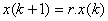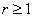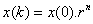## Geometric growth

Difference equation :Functional shortcut: ForCharacteristics:

• Multiply by a constant growth factor
• Grow with a fixed percentage or constant of growth factor in any period of a given length.

Below is an online interactive program to calculate Geometric growth at certain time n and plot the growth over time. Compare the plot with other growth type.

Initial value x(0) = Constant r = > 1 (must be larger or equal to one) Find the value of x at n =